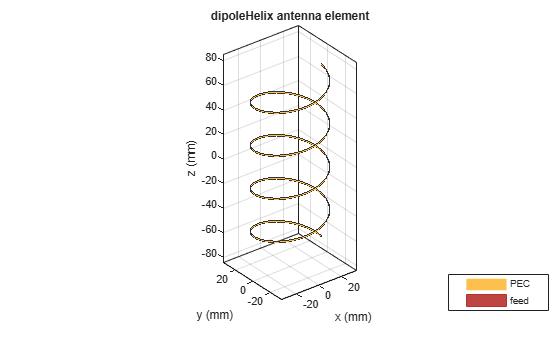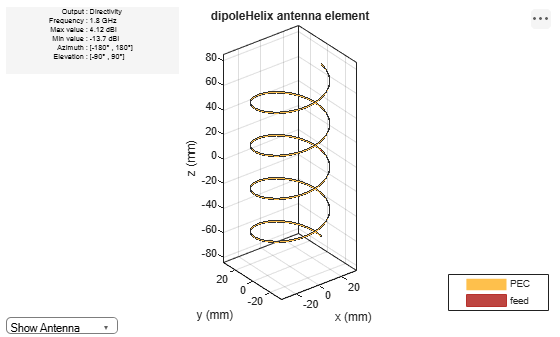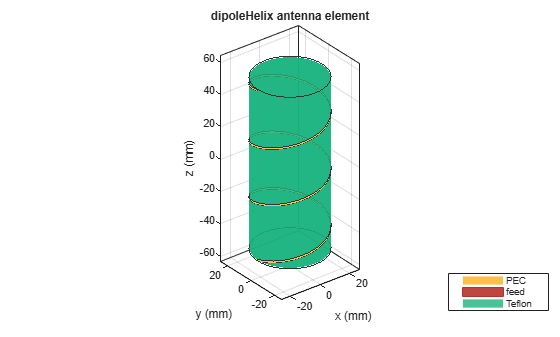# dipoleHelix

Create helical dipole antenna

## Description

The `dipoleHelix` object is a helical dipole antenna. The antenna is typically center-fed. You can move the feed along the antenna length using the feed offset property. Helical dipoles are used in satellite communications and wireless power transfers.

The width of the strip is related to the diameter of an equivalent cylinder by this equation

`$w=2d=4r$`

where:

• w is the width of the strip.

• d is the diameter of an equivalent cylinder.

• r is the radius of an equivalent cylinder.

For a given cylinder radius, use the `cylinder2strip` utility function to calculate the equivalent width. The default helical dipole antenna is center-fed. Commonly, helical dipole antennas are used in axial mode. In this mode, the helical dipole circumference is comparable to the operating wavelength, and has maximum directivity along its axis. In normal mode, the helical dipole radius is small compared to the operating wavelength. In this mode, the helical dipole radiates broadside, that is, in the plane perpendicular to its axis. The basic equation for the helical dipole antenna is:

$\begin{array}{l}x=r\mathrm{cos}\left(\theta \right)\\ y=r\mathrm{sin}\left(\theta \right)\\ z=S\theta \end{array}$

where:

• r is the radius of the helical dipole.

• θ is the winding angle.

• S is the spacing between turns.

For a given pitch angle in degrees, use the `helixpitch2spacing` utility function to calculate the spacing between the turns in meters.## Creation

### Syntax

``dh = dipoleHelix``
``dh = dipoleHelix(Name=Value)``

### Description

example

``` `dh = dipoleHelix` creates a helical dipole antenna. The default antenna operates at around 2 GHz.```

example

``` `dh = dipoleHelix(Name=Value)` sets Properties using one or more name–value arguments. `Name` is the property name and `Value` is the corresponding value. You can specify several name-value arguments in any order as `Name1=Value1`, `...`, `NameN=ValueN`. Properties that you do not specify retain their default values.```

## Properties

expand all

Turn radius, specified as a scalar in meters.

Example: `Radius=2`

Data Types: `double`

Strip width, specified as a scalar in meters.

Note

Strip width should be less than `'Radius'`/5 and greater than `'Radius'`/250. 

Example: `Width=5`

Data Types: `double`

Number of turns of the helical dipole, specified a scalar.

Example: `Turns=2`

Data Types: `double`

Spacing between turns, specified as a scalar in meters.

Example: `Spacing=1.5`

Data Types: `double`

Direction of helical dipole turns (windings), specified as `'CW'` or `'CCW'`.

Example: `WindingDirection='CW'`

Data Types: `char` | `string`

Type of dielectric material used as the substrate, specified as a `dielectric` object. You can specify only one dielectric layer in the `dipoleHelix` object. Specify the same radius for all the turns. When you use a dielectric material other than air, the number of turns in the dipole helix must be greater than 1. For more information on dielectric substrate meshing, see Meshing.

Example: `Substrate=dielectric('Teflon')`

Type of the metal used as a conductor, specified as a metal material object. You can choose any metal from the `MetalCatalog` or specify a metal of your choice. For more information, see `metal`. For more information on metal conductor meshing, see Meshing.

Example: `Conductor=metal('Copper');`

Lumped elements added to the antenna feed, specified as a lumped element object. For more information, see `lumpedElement`.

Example: `Load=lumpedElement(Impedance=75)`

Signed distance from center along length and width of ground plane, specified as a two-element vector in meters. Use this property to adjust the location of the feedpoint relative to the ground plane and patch.

Example: `FeedOffset=[0.01 0.01]`

Data Types: `double`

Tilt angle of the antenna, specified as a scalar or vector with each element unit in degrees. For more information, see Rotate Antennas and Arrays.

Example: `Tilt=90`

Example: `Tilt=[90 90]`,`TiltAxis=[0 1 0;0 1 1]` tilts the antenna at 90 degrees about the two axes defined by the vectors.

Note

The `wireStack` antenna object only accepts the dot method to change its properties.

Data Types: `double`

Tilt axis of the antenna, specified as:

• Three-element vector of Cartesian coordinates in meters. In this case, each coordinate in the vector starts at the origin and lies along the specified points on the X-, Y-, and Z-axes.

• Two points in space, each specified as three-element vectors of Cartesian coordinates. In this case, the antenna rotates around the line joining the two points in space.

• A string input describing simple rotations around one of the principal axes, 'X', 'Y', or 'Z'.

Example: `TiltAxis=[0 1 0]`

Example: `TiltAxis=[0 0 0;0 1 0]`

Example: `TiltAxis = 'Z'`

Data Types: `double`

## Object Functions

 `show` Display antenna, array structures or shapes `info` Display information about antenna or array `axialRatio` Axial ratio of antenna `beamwidth` Beamwidth of antenna `charge` Charge distribution on antenna or array surface `current` Current distribution on antenna or array surface `design` Design prototype antenna or arrays for resonance around specified frequency `efficiency` Radiation efficiency of antenna `EHfields` Electric and magnetic fields of antennas; Embedded electric and magnetic fields of antenna element in arrays `impedance` Input impedance of antenna; scan impedance of array `mesh` Mesh properties of metal, dielectric antenna, or array structure `meshconfig` Change mesh mode of antenna structure `optimize` Optimize antenna or array using SADEA optimizer `pattern` Radiation pattern and phase of antenna or array; Embedded pattern of antenna element in array `patternAzimuth` Azimuth pattern of antenna or array `patternElevation` Elevation pattern of antenna or array `rcs` Calculate and plot radar cross section (RCS) of platform, antenna, or array `returnLoss` Return loss of antenna; scan return loss of array `sparameters` Calculate S-parameter for antenna and antenna array objects `vswr` Voltage standing wave ratio of antenna

## Examples

collapse all

Create a default helical dipole antenna and view it.

`dh = dipoleHelix`
```dh = dipoleHelix with properties: Radius: 0.0220 Width: 1.0000e-03 Turns: 3 Spacing: 0.0350 WindingDirection: 'CCW' FeedOffset: 0 Substrate: [1x1 dielectric] Conductor: [1x1 metal] Tilt: 0 TiltAxis: [1 0 0] Load: [1x1 lumpedElement] ```
`show(dh)`Create a four-turn helical dipole antenna with a turn radius of 28 mm and a strip width of 1.2 mm.

```dh = dipoleHelix(Radius=28e-3, Width=1.2e-3, Turns=4); show(dh)```Plot the radiation pattern of the helical dipole at 1.8 GHz.

`pattern(dh, 1.8e9);`Create a custom dipole helix antenna with a Teflon dielectric substrate.

```d = dielectric('Teflon'); dh = dipoleHelix(Radius=22e-3,Width=1e-3,Turns=3,Spacing=35e-3,FeedOffset=0,Substrate=d)```
```dh = dipoleHelix with properties: Radius: 0.0220 Width: 1.0000e-03 Turns: 3 Spacing: 0.0350 WindingDirection: 'CCW' FeedOffset: 0 Substrate: [1x1 dielectric] Conductor: [1x1 metal] Tilt: 0 TiltAxis: [1 0 0] Load: [1x1 lumpedElement] ```

View the dipole helix antenna.

`show(dh)`Balanis, C. A. Antenna Theory. Analysis and Design. 3rd Ed. Hoboken, NJ: John Wiley & Sons, 2005.

 Volakis, John. Antenna Engineering Handbook. 4th Ed. New York: McGraw-Hill, 2007.